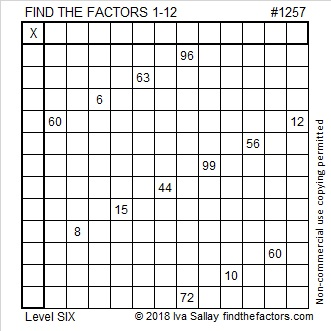# 1257 and Level 6

Both 6 and 12 are allowable common factors of 60 and 12. Likewise, both 8 and 12 are allowable common factors of 96 and 72. In each case, only one of those common factors will work with this puzzle. Don’t guess and check each one. Study the other clues and at least one wrong common factor will be eliminated. Have fun solving it!Print the puzzles or type the solution in this excel file: 12 factors 1251-1258

Now I’ll write a few things about the number 1257:

• 1257 is a composite number.
• Prime factorization: 1257 = 3 × 419
• The exponents in the prime factorization are 1 and 1. Adding one to each and multiplying we get (1 + 1)(1 + 1) = 2 × 2 = 4. Therefore 1257 has exactly 4 factors.
• Factors of 1257: 1, 3, 419, 1257
• Factor pairs: 1257 = 1 × 1257 or 3 × 419
• 1257 has no square factors that allow its square root to be simplified. √1257 ≈ 35.45421257 is the difference of two squares two different ways:
211² – 208² = 1257
629² – 628² = 1257

1257 is palindrome 393 in BASE 19

This site uses Akismet to reduce spam. Learn how your comment data is processed.